Linear Momentum and Collisions

# Introduction to Rocket Propulsion

OpenStaxCollege

### Learning Objectives

• State Newton’s third law of motion.
• Explain the principle involved in propulsion of rockets and jet engines.
• Derive an expression for the acceleration of the rocket and discuss the factors that affect the acceleration.
• Describe the function of a space shuttle.

Rockets range in size from fireworks so small that ordinary people use them to immense Saturn Vs that once propelled massive payloads toward the Moon. The propulsion of all rockets, jet engines, deflating balloons, and even squids and octopuses is explained by the same physical principle—Newton’s third law of motion. Matter is forcefully ejected from a system, producing an equal and opposite reaction on what remains. Another common example is the recoil of a gun. The gun exerts a force on a bullet to accelerate it and consequently experiences an equal and opposite force, causing the gun’s recoil or kick.

Making Connections: Take-Home Experiment—Propulsion of a Balloon

Hold a balloon and fill it with air. Then, let the balloon go. In which direction does the air come out of the balloon and in which direction does the balloon get propelled? If you fill the balloon with water and then let the balloon go, does the balloon’s direction change? Explain your answer.

[link] shows a rocket accelerating straight up. In part (a), the rocket has a mass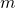and a velocity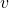relative to Earth, and hence a momentum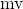. In part (b), a timehas elapsed in which the rocket has ejected a massof hot gas at a velocity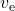relative to the rocket. The remainder of the mass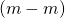now has a greater velocity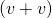. The momentum of the entire system (rocket plus expelled gas) has actually decreased because the force of gravity has acted for a time, producing a negative impulse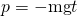. (Remember that impulse is the net external force on a system multiplied by the time it acts, and it equals the change in momentum of the system.) So, the center of mass of the system is in free fall but, by rapidly expelling mass, part of the system can accelerate upward. It is a commonly held misconception that the rocket exhaust pushes on the ground. If we consider thrust; that is, the force exerted on the rocket by the exhaust gases, then a rocket’s thrust is greater in outer space than in the atmosphere or on the launch pad. In fact, gases are easier to expel into a vacuum.

By calculating the change in momentum for the entire system over, and equating this change to the impulse, the following expression can be shown to be a good approximation for the acceleration of the rocket.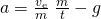“The rocket” is that part of the system remaining after the gas is ejected, and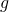is the acceleration due to gravity.

Acceleration of a Rocket

Acceleration of a rocket is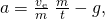where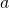is the acceleration of the rocket,is the escape velocity,is the mass of the rocket,is the mass of the ejected gas, andis the time in which the gas is ejected.

(a) This rocket has a massand an upward velocity. The net external force on the system is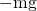, if air resistance is neglected. (b) A timelater the system has two main parts, the ejected gas and the remainder of the rocket. The reaction force on the rocket is what overcomes the gravitational force and accelerates it upward.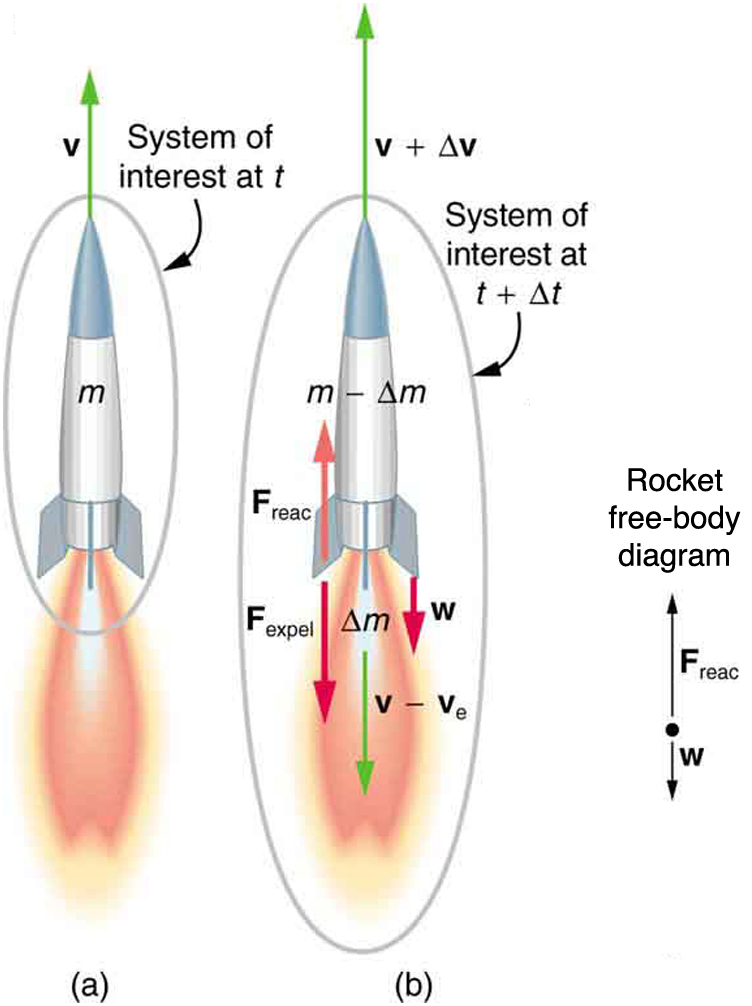A rocket’s acceleration depends on three major factors, consistent with the equation for acceleration of a rocket . First, the greater the exhaust velocity of the gases relative to the rocket,, the greater the acceleration is. The practical limit foris about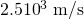for conventional (non-nuclear) hot-gas propulsion systems. The second factor is the rate at which mass is ejected from the rocket. This is the factor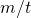in the equation. The quantity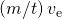, with units of newtons, is called “thrust.” The faster the rocket burns its fuel, the greater its thrust, and the greater its acceleration. The third factor is the massof the rocket. The smaller the mass is (all other factors being the same), the greater the acceleration. The rocket massdecreases dramatically during flight because most of the rocket is fuel to begin with, so that acceleration increases continuously, reaching a maximum just before the fuel is exhausted.

Factors Affecting a Rocket’s Acceleration
• The greater the exhaust velocityof the gases relative to the rocket, the greater the acceleration.
• The faster the rocket burns its fuel, the greater its acceleration.
• The smaller the rocket’s mass (all other factors being the same), the greater the acceleration.
Calculating Acceleration: Initial Acceleration of a Moon Launch

A Saturn V’s mass at liftoff was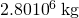, its fuel-burn rate was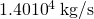, and the exhaust velocity was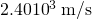. Calculate its initial acceleration.

Strategy

This problem is a straightforward application of the expression for acceleration becauseis the unknown and all of the terms on the right side of the equation are given.

Solution

Substituting the given values into the equation for acceleration yields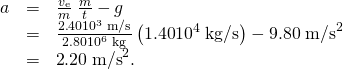Discussion

This value is fairly small, even for an initial acceleration. The acceleration does increase steadily as the rocket burns fuel, becausedecreases whileand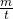remain constant. Knowing this acceleration and the mass of the rocket, you can show that the thrust of the engines was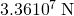.

To achieve the high speeds needed to hop continents, obtain orbit, or escape Earth’s gravity altogether, the mass of the rocket other than fuel must be as small as possible. It can be shown that, in the absence of air resistance and neglecting gravity, the final velocity of a one-stage rocket initially at rest is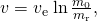where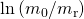is the natural logarithm of the ratio of the initial mass of the rocketto what is left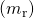after all of the fuel is exhausted. (Note thatis actually the change in velocity, so the equation can be used for any segment of the flight. If we start from rest, the change in velocity equals the final velocity.) For example, let us calculate the mass ratio needed to escape Earth’s gravity starting from rest, given that the escape velocity from Earth is about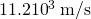, and assuming an exhaust velocity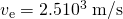.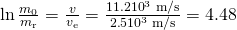Solving for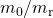gives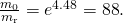Thus, the mass of the rocket is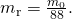This result means that only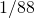of the mass is left when the fuel is burnt, and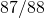of the initial mass was fuel. Expressed as percentages, 98.9% of the rocket is fuel, while payload, engines, fuel tanks, and other components make up only 1.10%. Taking air resistance and gravitational force into account, the mass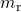remaining can only be about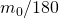. It is difficult to build a rocket in which the fuel has a mass 180 times everything else. The solution is multistage rockets. Each stage only needs to achieve part of the final velocity and is discarded after it burns its fuel. The result is that each successive stage can have smaller engines and more payload relative to its fuel. Once out of the atmosphere, the ratio of payload to fuel becomes more favorable, too.

The space shuttle was an attempt at an economical vehicle with some reusable parts, such as the solid fuel boosters and the craft itself. (See [link]) The shuttle’s need to be operated by humans, however, made it at least as costly for launching satellites as expendable, unmanned rockets. Ideally, the shuttle would only have been used when human activities were required for the success of a mission, such as the repair of the Hubble space telescope. Rockets with satellites can also be launched from airplanes. Using airplanes has the double advantage that the initial velocity is significantly above zero and a rocket can avoid most of the atmosphere’s resistance.

The space shuttle had a number of reusable parts. Solid fuel boosters on either side were recovered and refueled after each flight, and the entire orbiter returned to Earth for use in subsequent flights. The large liquid fuel tank was expended. The space shuttle was a complex assemblage of technologies, employing both solid and liquid fuel and pioneering ceramic tiles as reentry heat shields. As a result, it permitted multiple launches as opposed to single-use rockets. (credit: NASA)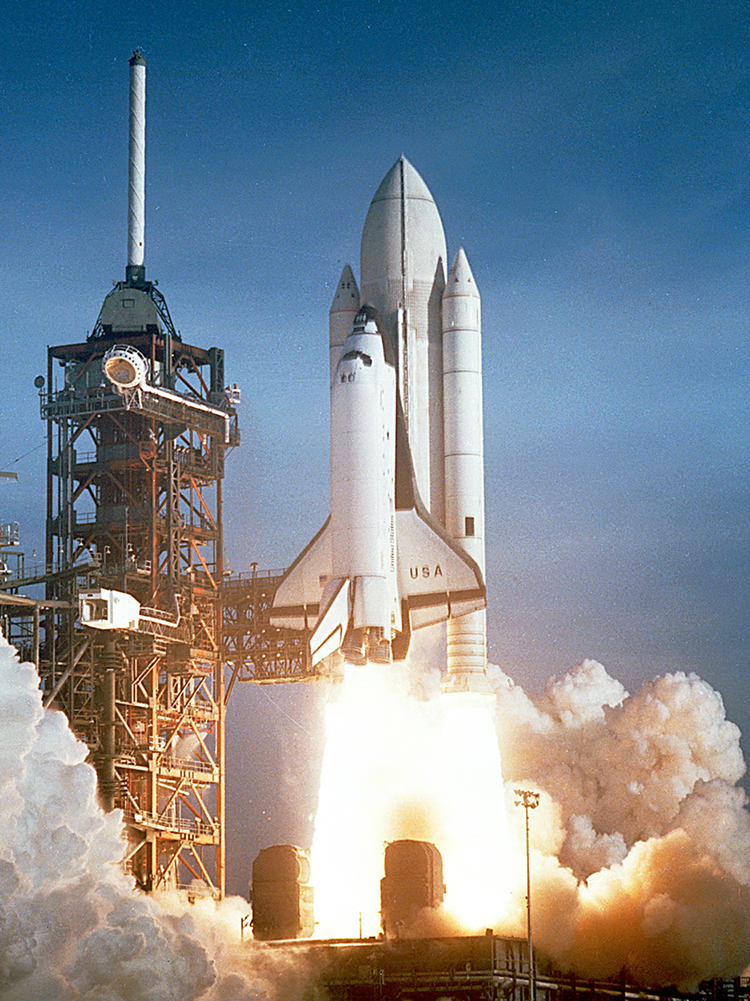PhET Explorations: Lunar Lander

Can you avoid the boulder field and land safely, just before your fuel runs out, as Neil Armstrong did in 1969? Our version of this classic video game accurately simulates the real motion of the lunar lander with the correct mass, thrust, fuel consumption rate, and lunar gravity. The real lunar lander is very hard to control.

# Section Summary

• Newton’s third law of motion states that to every action, there is an equal and opposite reaction.
• Acceleration of a rocket is.
• A rocket’s acceleration depends on three main factors. They are
1. The greater the exhaust velocity of the gases, the greater the acceleration.
2. The faster the rocket burns its fuel, the greater its acceleration.
3. The smaller the rocket’s mass, the greater the acceleration.

# Conceptual Questions

Professional Application

Suppose a fireworks shell explodes, breaking into three large pieces for which air resistance is negligible. How is the motion of the center of mass affected by the explosion? How would it be affected if the pieces experienced significantly more air resistance than the intact shell?

Professional Application

During a visit to the International Space Station, an astronaut was positioned motionless in the center of the station, out of reach of any solid object on which he could exert a force. Suggest a method by which he could move himself away from this position, and explain the physics involved.

Professional Application

It is possible for the velocity of a rocket to be greater than the exhaust velocity of the gases it ejects. When that is the case, the gas velocity and gas momentum are in the same direction as that of the rocket. How is the rocket still able to obtain thrust by ejecting the gases?

# Problems & Exercises

Professional Application

Antiballistic missiles (ABMs) are designed to have very large accelerations so that they may intercept fast-moving incoming missiles in the short time available. What is the takeoff acceleration of a 10,000-kg ABM that expels 196 kg of gas per second at an exhaust velocity of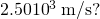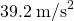Professional Application

What is the acceleration of a 5000-kg rocket taking off from the Moon, where the acceleration due to gravity is only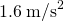, if the rocket expels 8.00 kg of gas per second at an exhaust velocity of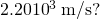Professional Application

Calculate the increase in velocity of a 4000-kg space probe that expels 3500 kg of its mass at an exhaust velocity of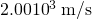. You may assume the gravitational force is negligible at the probe’s location.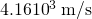Professional Application

Ion-propulsion rockets have been proposed for use in space. They employ atomic ionization techniques and nuclear energy sources to produce extremely high exhaust velocities, perhaps as great as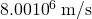. These techniques allow a much more favorable payload-to-fuel ratio. To illustrate this fact: (a) Calculate the increase in velocity of a 20,000-kg space probe that expels only 40.0-kg of its mass at the given exhaust velocity. (b) These engines are usually designed to produce a very small thrust for a very long time—the type of engine that might be useful on a trip to the outer planets, for example. Calculate the acceleration of such an engine if it expels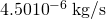at the given velocity, assuming the acceleration due to gravity is negligible.

Derive the equation for the vertical acceleration of a rocket.

The force needed to give a small massan acceleration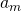is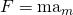. To accelerate this mass in the small time intervalat a speedrequires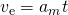, so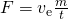. By Newton’s third law, this force is equal in magnitude to the thrust force acting on the rocket, so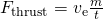, where all quantities are positive. Applying Newton’s second law to the rocket gives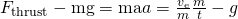, whereis the mass of the rocket and unburnt fuel.

Professional Application

(a) Calculate the maximum rate at which a rocket can expel gases if its acceleration cannot exceed seven times that of gravity. The mass of the rocket just as it runs out of fuel is 75,000-kg, and its exhaust velocity is. Assume that the acceleration of gravity is the same as on Earth’s surface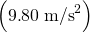. (b) Why might it be necessary to limit the acceleration of a rocket?

Given the following data for a fire extinguisher-toy wagon rocket experiment, calculate the average exhaust velocity of the gases expelled from the extinguisher. Starting from rest, the final velocity is 10.0 m/s. The total mass is initially 75.0 kg and is 70.0 kg after the extinguisher is fired.

How much of a single-stage rocket that is 100,000 kg can be anything but fuel if the rocket is to have a final speed of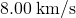, given that it expels gases at an exhaust velocity of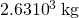Professional Application

(a) A 5.00-kg squid initially at rest ejects 0.250-kg of fluid with a velocity of 10.0 m/s. What is the recoil velocity of the squid if the ejection is done in 0.100 s and there is a 5.00-N frictional force opposing the squid’s movement. (b) How much energy is lost to work done against friction?

(a) 0.421 m/s away from the ejected fluid.

(b)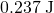.

Unreasonable Results

Squids have been reported to jump from the ocean and travel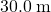(measured horizontally) before re-entering the water. (a) Calculate the initial speed of the squid if it leaves the water at an angle of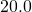, assuming negligible lift from the air and negligible air resistance. (b) The squid propels itself by squirting water. What fraction of its mass would it have to eject in order to achieve the speed found in the previous part? The water is ejected at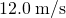; gravitational force and friction are neglected. (c) What is unreasonable about the results? (d) Which premise is unreasonable, or which premises are inconsistent?

Construct Your Own Problem

Consider an astronaut in deep space cut free from her space ship and needing to get back to it. The astronaut has a few packages that she can throw away to move herself toward the ship. Construct a problem in which you calculate the time it takes her to get back by throwing all the packages at one time compared to throwing them one at a time. Among the things to be considered are the masses involved, the force she can exert on the packages through some distance, and the distance to the ship.

Construct Your Own Problem

Consider an artillery projectile striking armor plating. Construct a problem in which you find the force exerted by the projectile on the plate. Among the things to be considered are the mass and speed of the projectile and the distance over which its speed is reduced. Your instructor may also wish for you to consider the relative merits of depleted uranium versus lead projectiles based on the greater density of uranium.

## LicenseIntroduction to Rocket Propulsion by OpenStaxCollege is licensed under a Creative Commons Attribution 4.0 International License, except where otherwise noted.